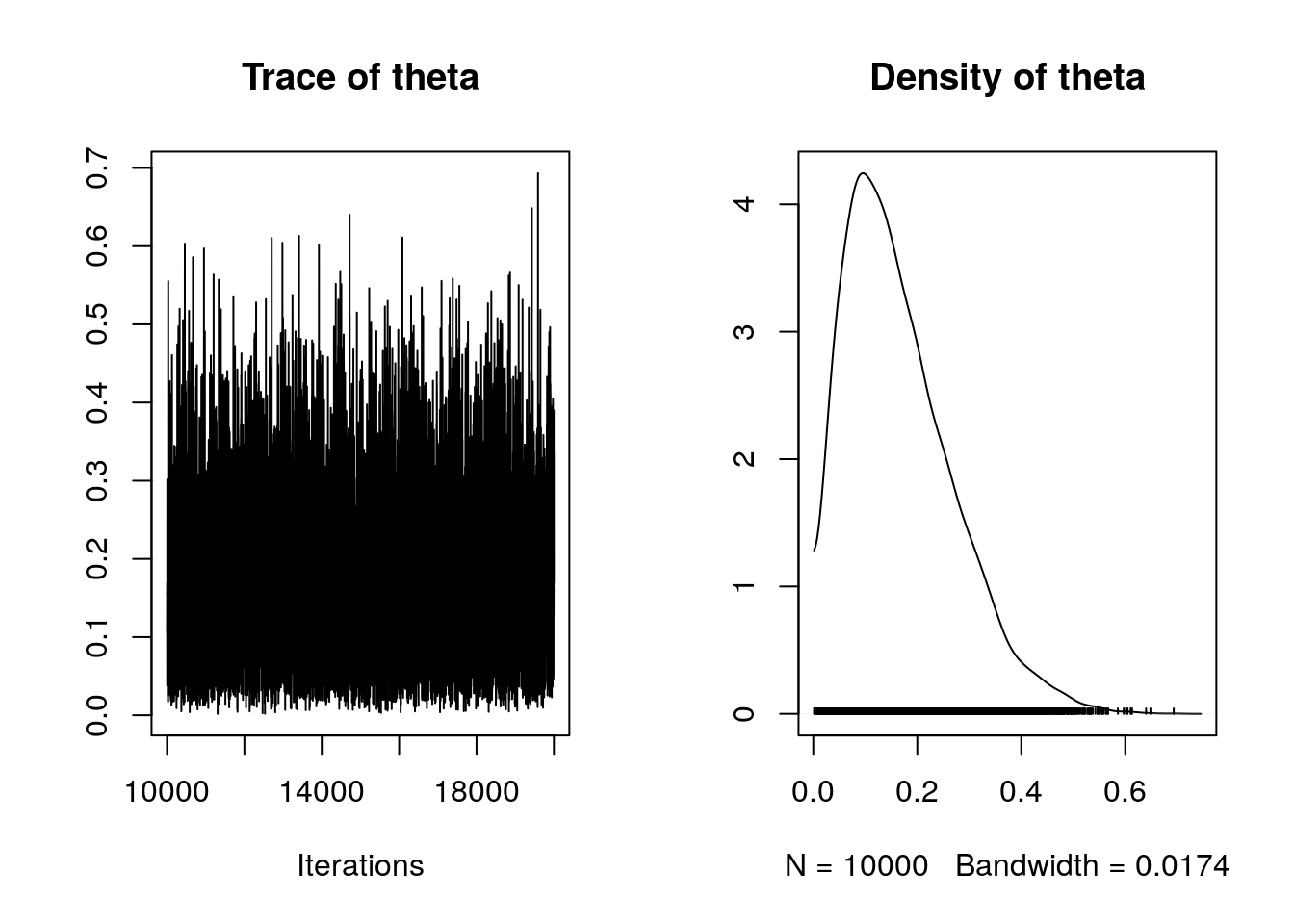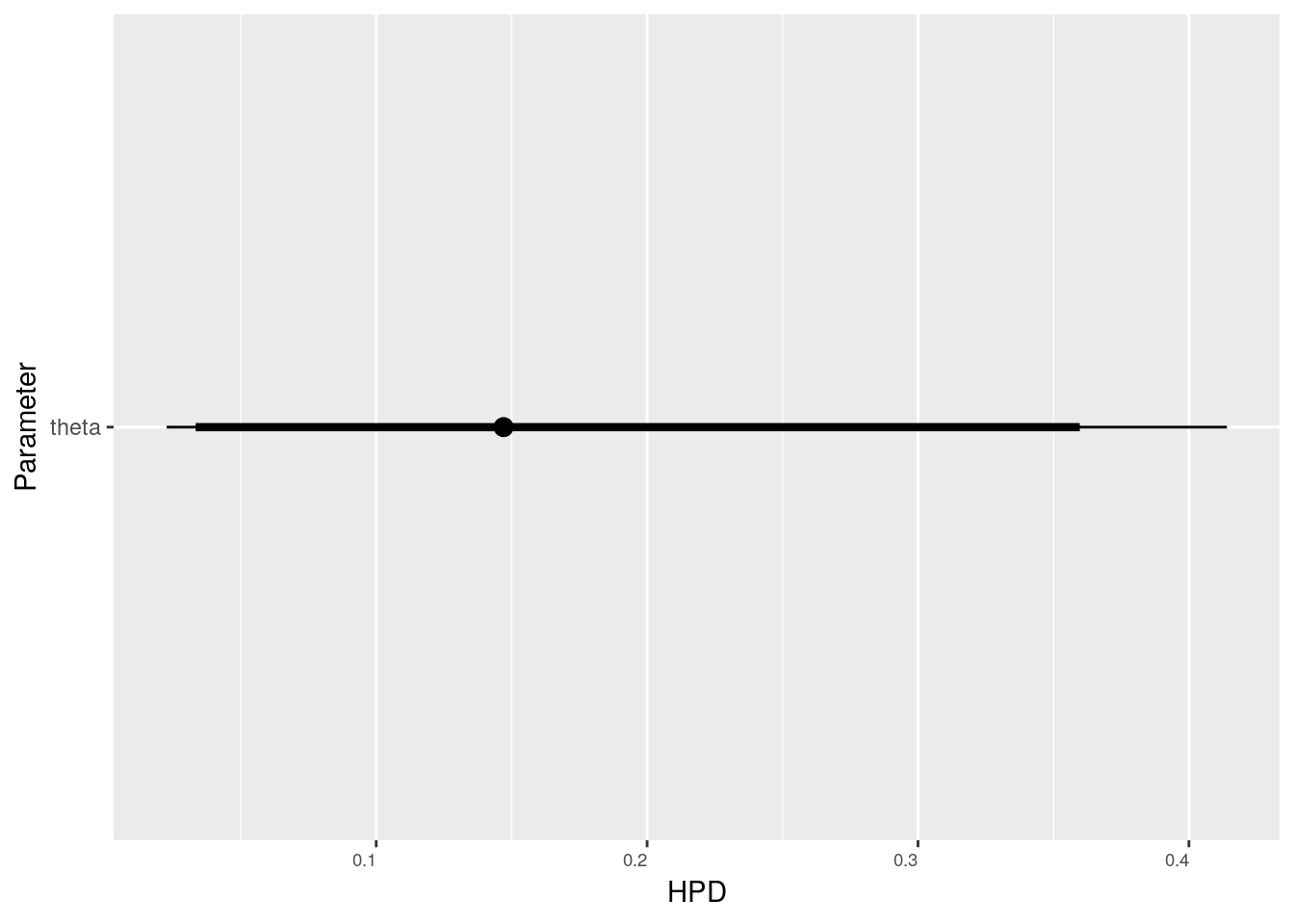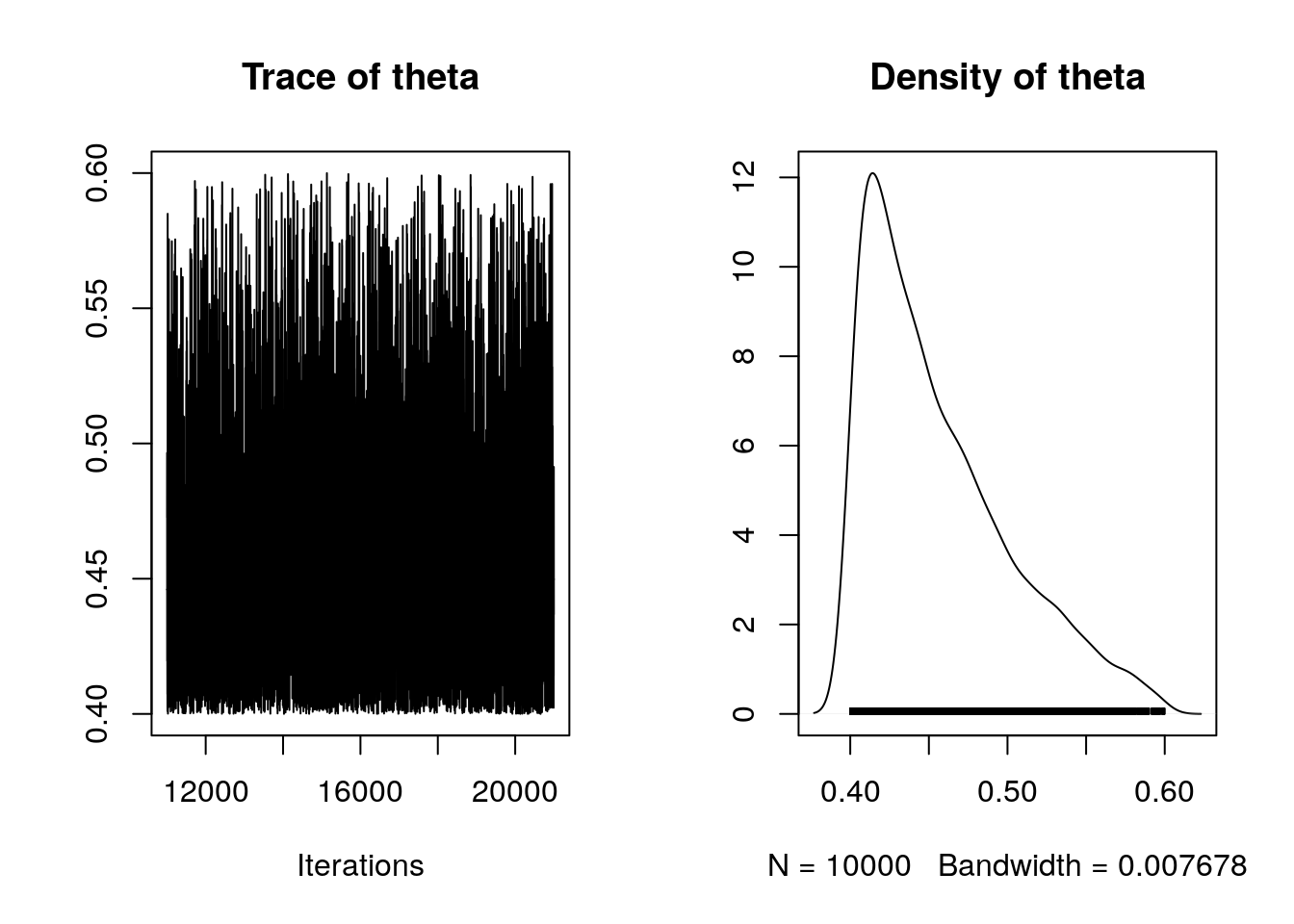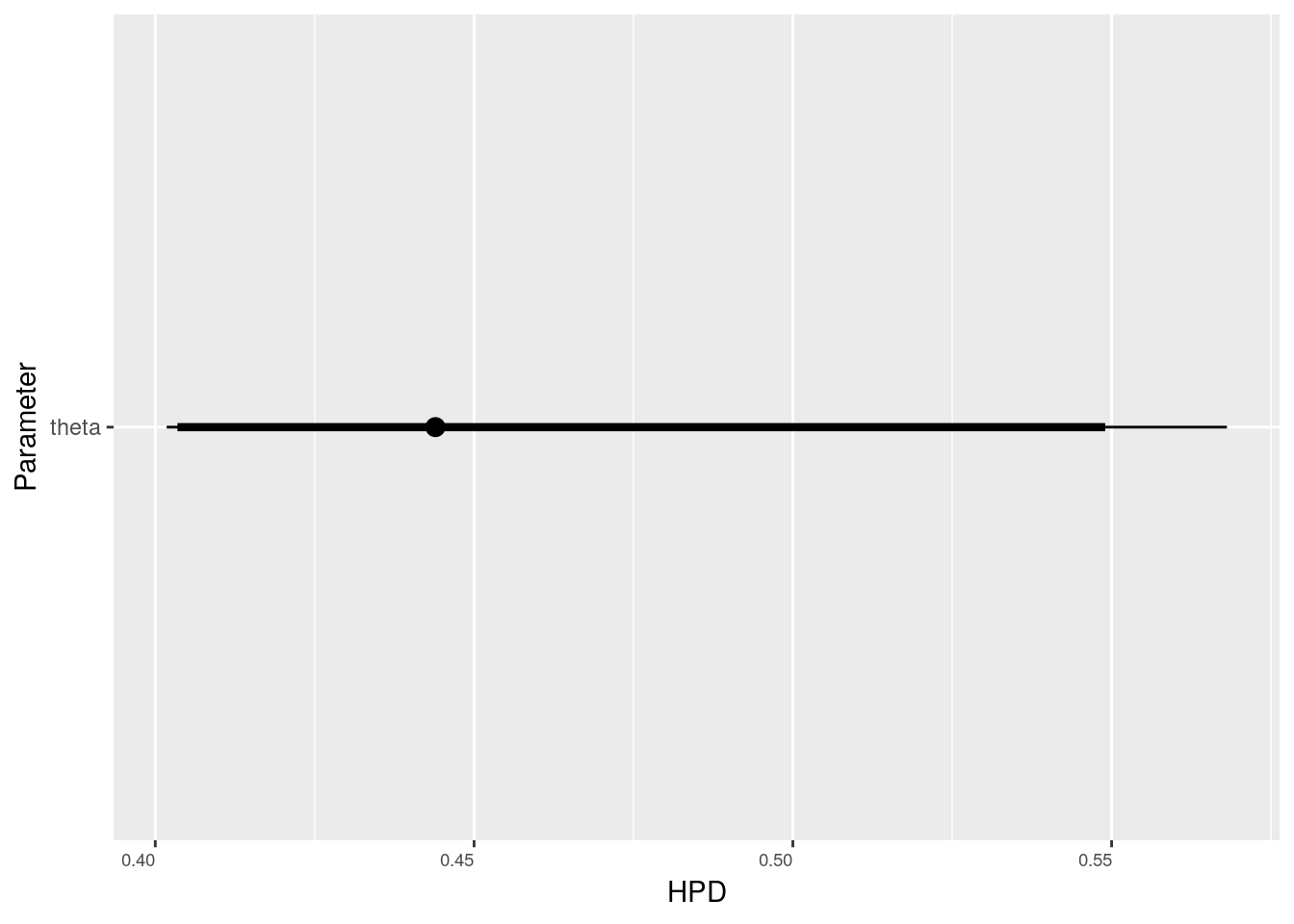## Introduction

Once you get used to the logic JAGS is a great tool for conducting simple thought experiments to help understand the logic behind Bayesian inference.

A very simple, classic example. Set up a model for benouli trials with uniform prior.

OK. In layman’s terms this is the coin flip example. Heads or tails. The bias on the coin takes a uniform prior between a low and a high value.

library(rjags)
library(tidyverse)
library(ggmcmc)

coin_toss<-"model {
for (i in 1:N){
x[i] ~ dbern(theta)
}
theta ~ dunif(low,high)

}"

## Improbable coin tosses

Say we observe nine heads and one tail from ten tosses. If we assume that all coins can potentially be biased and that the degree of the bias is considered to be unform between pure trick coins that throw only heads or only tails and everything in between. In this unusual situation then any inference we can make involving how biased the coin is will be based on purely on the likihood of the data.

x<-c(0,0,0,0,0,0,0,0,0,1)
N<-length(x)

dat<-list(
low=0,
high=1,
x=x,
N=N
)

model <- jags.model(textConnection(coin_toss),
data = dat,
n.chains=1)
update(model, 10000)
mod_sim <- coda.samples(model = model, variable.names = c("theta"), n.iter = 10000)
summary(mod_sim)
##
## Iterations = 10001:20000
## Thinning interval = 1
## Number of chains = 1
## Sample size per chain = 10000
##
## 1. Empirical mean and standard deviation for each variable,
##    plus standard error of the mean:
##
##           Mean             SD       Naive SE Time-series SE
##       0.166291       0.103579       0.001036       0.001036
##
## 2. Quantiles for each variable:
##
##    2.5%     25%     50%     75%   97.5%
## 0.02266 0.08662 0.14702 0.22808 0.41403
plot(mod_sim)So apparently the most likely value for the coin’s bias equates to the proportion of heads thrown.

## Highest posterior density

Does the HPD contain 0.5 ? The answer is no. So conventionally we can reject the hypothesis of a fair coin.

ms <-ggs(mod_sim)
ggs_caterpillar(ms)## What if coins are only likely to be slightly biased?

This shows up the problem when uninformative priors are used uncritically. In the above example we could now effectively rule out the probability that the coin was fair. However common sense dictates that even if real coins are biased, they are not likely to be uniformly biased in such an extreme way. What if bent coins may be about 10% biased around a fair toss. Is our coin now likely to be fair?

Let’s set the uniform prior to lie between 0.4 and 0.6 and repeat the model sampling.

x<-c(0,0,0,0,0,0,0,0,0,1)
N<-length(x)

dat<-list(
low=0.4,
high=0.6,
x=x,
N=N
)

model <- jags.model(textConnection(coin_toss),
data = dat,
n.chains=1)
update(model, 10000)
mod_sim <- coda.samples(model = model, variable.names = c("theta"), n.iter = 10000)

Now, the distribution is skewed, i.e. the most probable value for the coin’s bias does lie heavily on the left hand side of the distribution (0.4). However we now can’t rule out the probability that the coin was in fact actually fair.

summary(mod_sim)
##
## Iterations = 11001:21000
## Thinning interval = 1
## Number of chains = 1
## Sample size per chain = 10000
##
## 1. Empirical mean and standard deviation for each variable,
##    plus standard error of the mean:
##
##           Mean             SD       Naive SE Time-series SE
##      0.4559740      0.0457017      0.0004570      0.0008451
##
## 2. Quantiles for each variable:
##
##   2.5%    25%    50%    75%  97.5%
## 0.4018 0.4189 0.4439 0.4829 0.5681
plot(mod_sim)## Highest posterior density

Does the HPD contain 0.5 now? The answer is yes. So conventionally we can not reject the hypothesis of a fair coin, even though the balance of probablities still suggest that the coin may be biased in favour of heads.

ms <-ggs(mod_sim)
ggs_caterpillar(ms)## Moral of the story

Onserving an improbable sequence of coin tosses could be used to test multiple hypotheses. In this case it is assumed that a coin has bee drawn from some fictitious population of potentially biased coins. However the person tossing the coin could simply be a cheat, or a magician. In such a case some prior knowledge of the skill at cheating or the persons magical powers will be required.

If we do propose any hypothesis to explain an unlikely event then the only situation in which the likelihood of the data given all the possible values of the parameter will equal the distribution of the parameter itself is when all possible outcomes are equally likely. Using sharp cut offs on uniform distributions, as done here, is usually a far too brutal approach for practical analytical purposes. However it simply demonstrate the key principle that the probability of the hypothesis given the data is not the same as the probability of the data given the hypothesis. A uniform prior is just an assumption like any other assumption, and in many cases may be a rather poor one.